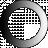# American Dictionary of the English Language

Dictionary Search

### Calculate

CALCULATE, verb transitive

1. To compute; to reckon; to add, subtract, multiply or divide any sums, for the purpose of finding the amount, difference, or other result. This, to calculate the expenses of erecting a house, is to estimate and add together the several sums which each part of the materials and the work will cost.

2. To ascertain by the use of tables or numbers; as, to calculate an eclipse.

3. To form tables upon mathematical principles, as logarithms, ephemerides, etc.

4. To compute the situation of the planets at a certain time, for astrological purposes; as, to calculate the birth of a person.

5. To adjust by computation; to fit or prepare by the adaptation of the means to the end; as, to calculate a system of laws for a free people. Religion is calculated for our benefit.

CALCULATE, verb intransitive To make a computation; as, we calculate better for ourselves than for others.

In popular use, this word is often equivalent to intend or purpose, that is, to make arrangements, and form a plan; as, a man calculated to go a journey. This use of the word springs from the practice of computing or estimating the various circumstances which concur to influence the mind in forming its determinations.